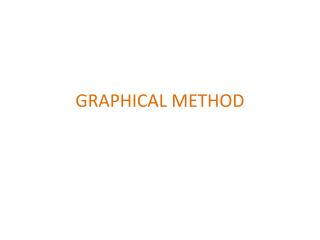DownloadDownload PresentationGRAPHICAL METHOD

# GRAPHICAL METHOD

Télécharger la présentation## GRAPHICAL METHOD

- - - - - - - - - - - - - - - - - - - - - - - - - - - E N D - - - - - - - - - - - - - - - - - - - - - - - - - - -
##### Presentation Transcript

1. GRAPHICAL METHOD

2. Graphical method can be applied to LPP with two variables. • The entire set of feasible solution( feasible region) can be displayed graphically by plotting linear constraints on a graph paper to locate the optimal solution.

3. DEFINITIONS • Solution is the set of values of decision variables(x1 , x2…. xn) which satisfy the constraints of an LP problem . • Feasible Solution is a solution for which all the constraints are satisfied. • Infeasible Solution is a solution for which atleast one constraint is violated. • Feasible Region is the collection of all feasible solutions.

4. Optimal Solution is a feasible solution that has the most favourablevalue of the objective function. • Most favourable value is the largest value if the objective function is to be maximised, and is the smallest value if the objective function is to be minimized. • Corner-Point feasible (CPF) solution is a solution that lies at a corner of the feasible region.

5. PROCEDURE • Write the problem in mathematical form • Write the inequality constraints as equality constraints. • Draw the graph. • Identify the feasible region or solution space. • Locate the corner points or vertices of the feasible region. • Evaluate the objective function at the corner points . • Find the optimum value of the objective function.

6. Multiple optimal solution : LPP attains optimality at more than one corner point of the feasible region. • Unbounded Solution : One or more decision variable will increase indefinitely without violating feasibility and thus the value of the objective function can be increased or decreased indefinitely. • Infeasible Solution ( no solution) : All the constraints cannot be satisfied simultaneously.